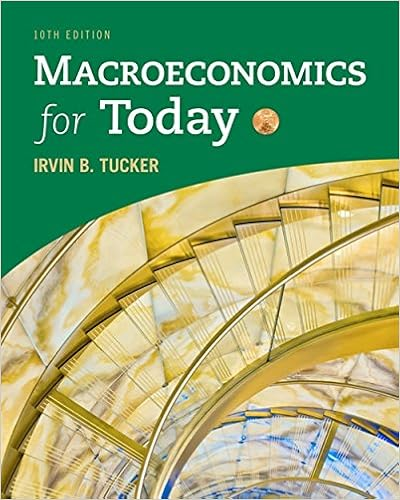# In 2002 the gdp deflator for old york was 300 and in

• Notes
• harrynn
• 17
• 100% (13) 13 out of 13 people found this document helpful

This preview shows page 12 - 15 out of 17 pages.

##### We have textbook solutions for you!
The document you are viewing contains questions related to this textbook.The document you are viewing contains questions related to this textbook.
Chapter 7 / Exercise 17
Macroeconomics for Today
TuckerExpert Verified
49.In 2002 the GDP deflator for Old York was 300, and in 2004 it had risen to 330.75. Based on thisinformation the annual average inflation rate for the two years was(a) 5%.(b) 5.125%.(c) 10%.(d) 10.25%.Answer: A
Level of difficulty: 1Section: 2.450.If the price index last year was 1.0 and today it is 1.4, what is the inflation rate over this period?
Level of difficulty: 1Section: 2.451.You are given information on the consumer price index (CPI), where the values given are those forDecember 31 of each year.Year CPI19891990199119921993126.1133.8137.9141.9145.8In which year was the inflation rate the highest?
Level of difficulty: 2Section: 2.4
##### We have textbook solutions for you!
The document you are viewing contains questions related to this textbook.The document you are viewing contains questions related to this textbook.
Chapter 7 / Exercise 17
Macroeconomics for Today
TuckerExpert Verified
26Abel/Bernanke • Macroeconomics, Fifth Edition52.The consumer price index (CPI) was 180 for 2002 when using 1983 as the base year (1983 =100).Now suppose we switch and use 2002 as the base year (2002 =100). What is the CPI for 1983 withthe new base year?
Level of difficulty: 3Section: 2.453.The Boskin Commission concluded that the CPI overstates increases in the cost of living by___________ percentage point(s) per year.(a) Less than 1(b) 1 to 2(c) About 3(d) Over 4Answer: B
Level of difficulty: 2Section: 2.454.The CPI may overstate inflation for all the following reasons EXCEPT
Level of difficulty: 1Section: 2.455.The nominal interest rate minus the inflation rate is the
Level of difficulty: 1Section: 2.556.By Marks buys a one-year German government bond (called a bund) for \$400. He receives principaland interest totaling \$436 one year later. During the year the CPI rose from 150 to 162. The nominalinterest rate on the bond was ___________, and the real interest rate was ___________.
Level of difficulty: 2Section: 2.5
Chapter 2The Measurement and Structure of the National Economy2757.The expected real interest rate (r) is equal to(a) nominal interest rate minus inflation rate.(b) nominal interest rate minus expected inflation rate.(c) expected nominal interest rate minus inflation rate.(d) nominal interest rate plus expected inflation rate.Answer: B
Level of difficulty: 1Section: 2.558.By Marks buys a one-year German government bond (called a bund) for \$400. He receives principaland interest totaling \$436 one year later. During the year the CPI rose from 150 to 162, but he hadthought the CPI would be at 159 by the end of the year. By Marks had expected the real interest rateto be ___________, but it actually turned out to be ___________.
Level of difficulty: 3Section: 2.5
•••